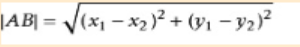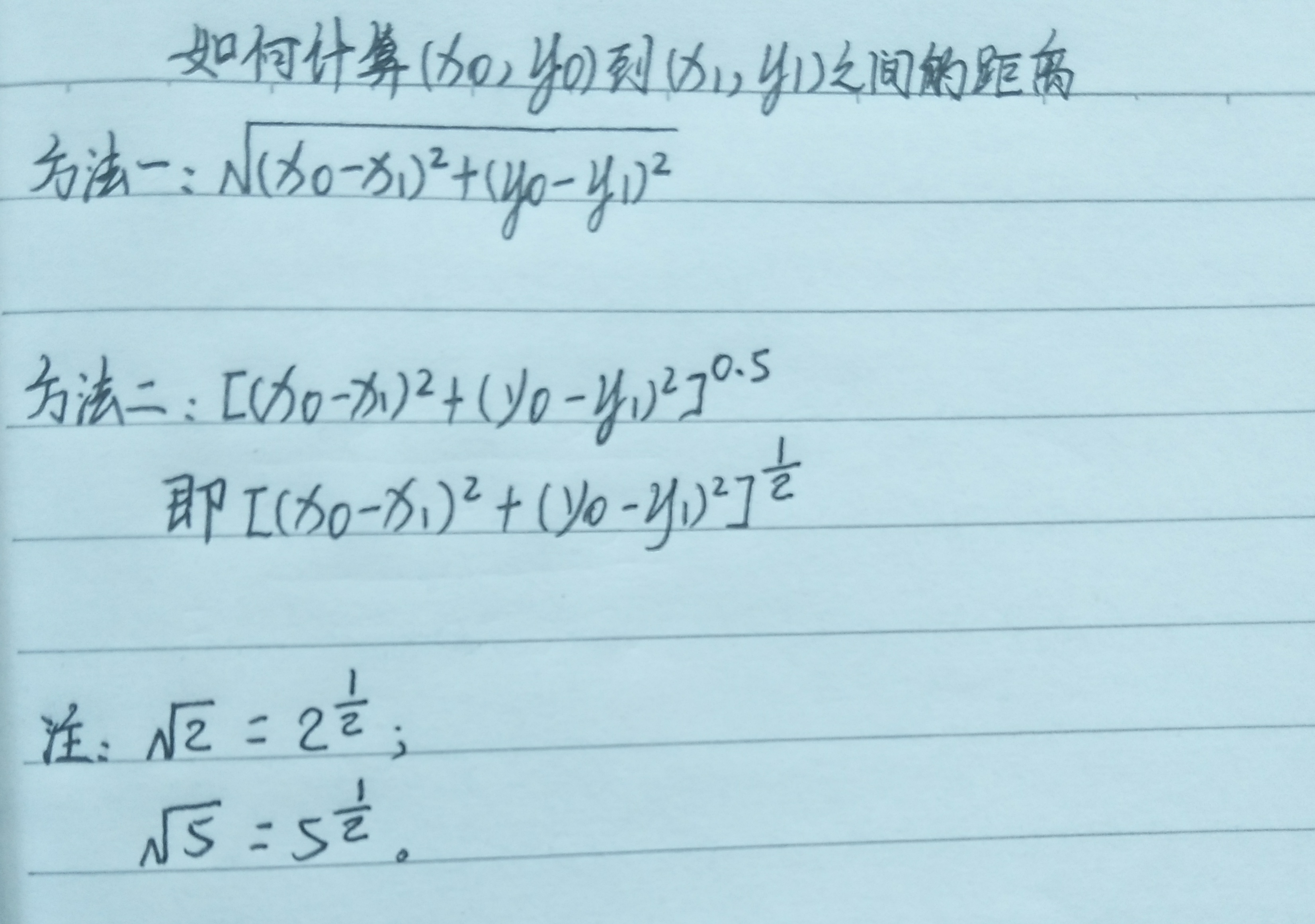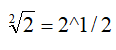• python 计算两点之间距离
2022-04-04 12:20:12

已知两个点的经度和纬度，计算两个点之间的距离

import pyproj

lat1,long1 = (37.8101,-122.4105)
lat2,long2 = (37.8024,-122.4058)

geod = pyproj.Geod(ellps="WGS84")

angle1,angle2, distance = geod.inv(long1,lat1,long2,lat2)

print(format(distance))


该例子来自《Python Geospatial Development》一书，此处仅作为学习笔记。

更多相关内容
• 今天小编就为大家分享一篇Python两点之间的直线距离(2种实现方法)，具有很好的参考价值，希望对大家有所帮助。一起跟随小编过来看看吧
• 计算两点(x1,y1),(x2,y2)之间距离，输出并保留一位小数 两点之间距离的数学公式： txt=input("请输入四个数字，用空格隔开:") ls=txt.split(" ") x1=eval(ls) y1=eval(ls) x2=eval(ls) y2=eval(ls) r...

从键盘输入4个数字，采用空格分隔，对应变量为x1,y1,x2,y2。计算两点(x1,y1),(x2,y2)之间的距离，输出并保留一位小数

两点之间距离的数学公式：txt=input("请输入四个数字，用空格隔开:")
ls=txt.split(" ")
x1=eval(ls)
y1=eval(ls)
x2=eval(ls)
y2=eval(ls)
r=pow(pow(x1-x2,2)+pow(y1-y2,2),0.5)
print("{:.1f}".format(r))展开全文开发语言 后端
• 今天小编就为大家分享一篇python计算两个地址之间距离方法，具有很好的参考价值，希望对大家有所帮助。一起跟随小编过来看看吧
• ## 用Python如何计算两点间距离

万次阅读 多人点赞 2020-03-02 17:27:36
Python如何计算两点间距离？ 第一步，我们先来看一下两点距离公式。 两点间距离公式： ？？？这是个啥？ 是不是方法一还能看懂，方法二却一脸懵逼？ 请仔细看注释， 也就是说，根号2还可以写成2的...

# 用Python如何计算两点间距离？

## 第一步，我们先来看一下两点间的距离公式。

### 两点间距离公式：## ？？？这是个啥？是不是方法一还能看懂，方法二却一脸懵逼？

## 请仔细看注释，### 也就是说，根号2还可以写成2的0.5(也就是1/2)次方

这个是高中学过的知识，不知道你有没有想起来，哈哈......

## 第二步，我们需要学习一下pow()这个函数

### pow(a,b)是计算a的b次方的一个函数

例如：pow(2,3)为2的3次方，为8

## 第三步，split()函数

### split()函数是将数据按指定格式分割开，默认为空格

例1：

a = "0 1 3 5"

print(a.slpit())

将a中的数据以空格的分割开，并返回一个['0', '1', '3', '5']形式的列表

例2：

a = 0,1,3,5

print(s.split(","))

将a中的数据以逗号分割开，并返回一个['0', '1', '3', '5']形式的列表

## 第四步 format()格式化

### format()格式化与%s, %d, %f有异曲同工之效，都是先为数据占一个位，而后补充数据

例1：

weekday = "星期一"

print('今天是%s' % weekday)

显示为：今天是星期一

例2：

weekday = "星期一"

print('今天是{}'.format(weekday))

### 第五步，我们看一下具体的代码~

从键盘上输入4个数字，各数字采用空格分隔，对应变量为x0, y0, x1, y1。计算两点(x0, y0)和(x1, y1)之间的距离。在屏幕上输出这个距离，保留2位小数。例如，键盘输入0 1 3 5，屏幕输出5.00

  #输入数据
ntxt=input('请输入4个数字(空格分隔):')
#利用split()函数，通过空格将数据分隔开并赋值给变量nls
nls=ntxt.split()
#利用eval()函数将字符串类型转化为数据类型；
#nls，将nls中的第零个数据赋值给变量x0
x0=eval(nls)
#nls，将nls中的第一个数据赋值给变量y0
y0=eval(nls)
#nls，将nls中的第二个数据赋值给变量x1
x1=eval(nls)
#nls，将nls中的第三个数据赋值给变量y1
y1=eval(nls)
#利用pow()函数计算两点间距离
r=pow(pow(x1-x0,2)+pow(y1-y0,2),0.5)
#打印数据；其中2f表示保留两位小数
print('{:.2f}'.format(r))

注：print('{:.2f}'.format(r))的意思是：用{:.2f}先占一个位置; .2f表示保留两位小数。

展开全文• 计算两点之间距离日常旁白：本人是一枚生物学的学生，由于对生物信息学特别感兴趣，于是想自学生物信息学（新手莫怪）。了解到生物信息学要有编程基础，尤其是要会一门编程语言，例如：R语言、Python、Perl等，...

计算出两点之间的距离

日常旁白：本人是一枚生物学的学生，由于对生物信息学特别感兴趣，于是想自学生物信息学（新手莫怪）。了解到生物信息学要有编程基础，尤其是要会一门编程语言，例如：R语言、Python、Perl等，还要熟悉Linux系统，作为生信小白，听说Python挺简单的，于是就自学了Python，花了两天时间了解了Python的基础语法后，今天想做个练习题试试手（实践是检验真理的唯一标准），下面是练习题：(试题来源：《PYTHON生物信息学数据管理》)试题02

书中答案原代码如下：

from math import *

x1,y1,z1 = 0.1,0.0,-0.7

x2,y2,z2 = 0.5,-1.0,2.7

dx = x1-x2

dy = y1-y2

dz = z1-z2

dsquare = pow(dx,2)+pow(dy,2)+pow(dz,2)

d = sqrt(dsquare)

print(d)

为了将所学内容活用，充分理解思路，特拆分讲解。（嫌麻烦的可以直接查看最后的升级版代码）

第一步：导入math模块

from math import *

第二步：获取两个点的坐标

①方便随时计算用户输入的两点的坐标并计算出两个点之间的距离。特增加了获取用户输入的input()函数。

#获取用户输入

x1,y1,z1=input("请依次输入点1纵横坐标的值：",)

x2,y2,z2=input("请依次输入点2纵横坐标的值：",)

②为了简化同时获取用户输入的点的坐标的三个值，使用了切片功能。split(',')表示以“，”作为分隔符，切割用户输入的三个字符。

x1,y1,z1=input("请依次输入点1纵横坐标的值：",).split(',')

x2,y2,z2=input("请依次输入点2纵横坐标的值：",).split(',')

第三步：编写计算公式

①计算差值

由于系统默认用户输入的是字符，所以使用下面的代码计算可能会报错

dx = x1-x2

dy = y1-y2

dz = z1-z2

因此增加了eval（）函数，将字符串转化为数值，方便下面的计算，代码如下：

dx = eval(x1) -eval(x2)

dy = eval(y1) -eval(y2)

dz = eval(z1) -eval(z2)

②计算公式

计算公式代码如下：

dsquare = pow(dx,2)+pow(dy,2)+pow(dz,2)

d = sqrt(dsquare)

第四步：输出结果

print（d）

最后将代码总结如下：

from math import *

#获取用户输入

x1,y1,z1=input("请依次输入点1纵横坐标的值：",).split(',')

x2,y2,z2=input("请依次输入点2纵横坐标的值：",).split(',')

dx = eval(x1) -eval(x2)

dy = eval(y1) -eval(y2)

dz = eval(z1) -eval(z2)

dsquare = pow(dx,2)+pow(dy,2)+pow(dz,2)

d = sqrt(dsquare)

print(d)

虽然这是个小小的计算程序，但对于初学者的我来说每一次对原代码的升级改造，哪怕是读懂后的注释都感觉是一次进步提升，总之代码虽小，动手最重要！希望更多学习Python的爱好者不要像我一样眼高手低，学习编程就是要，思考，敲码，思考，敲码，敲码，再敲码！

展开全文• 计算是在您提取的二维灰色矩阵上进行的。我已经为输入到cluster的矩阵的感兴趣的值指定了0和1，在本例中是KMeans，尽管可以根据需要用其他模型替换它。import cv2import numpy as npfilename = 'Dog.jpg'img = cv2....
• 如下所示： distances = np.sqrt(np.sum(np.asarray(airportPosition - x_vals)**2, axis=1)) ... 您可能感兴趣的文章:python计算两个地址之间距离方法Python实现的计算马氏距离算法示例Python实现计
• #coding:UTF-8 """ Python implementation of Haversine formula Copyright (C) <2009> Bartek Górny This program is free software: you can redistribute it and/or modify it under the terms of the GNU ...
• 算了，找了半天，就用第一种方法吧 dis = math.hypot(10,10) 计算和0,0之间距离
• 我需要创建一个列表,找到最接近的,然后打印出来.如何比较列表中的每个？没有任何需要绘制或任何东西,只是比较,找到列表中最接近的个.import math # 'math' needed for 'sqrt'# Distance functiondef ...
• My question is there a function or library for python? 解决方案from math import * def greatCircleDistance((lat1, lon1), (lat2, lon2)): def haversin(x): return sin(x/2)**2 return 2 * asin(sqrt( ...
• 角度检测 Python-OpenCV应用计算两点之间的角度 如果您打开该程序的LIVE版本，它将打开设置，在此处应管理所需颜色的HSV范围 如果您打开非实时版本，则应选择想要使用的图片
• from math import radians, cos, sin, asin, sqrt   def haversine(lon1, lat1, lon2, lat2): # 经度1，纬度1，经度2，纬度2   """  Calculate the great circle distance between two points  ...
• "用haversine公式计算球面两点间距离。" # 经纬度转换成弧度 lat0 = radians(lat0) lat1 = radians(lat1) lng0 = radians(lng0) lng1 = radians(lng1) dlng = fabs(lng0 - lng1) dlat = fabs(lat0 - lat1...
• 太难找了，自己写个博客记录一下 hypot()方法返回的欧几里德范数 sqrt(xx + yy). ...len = hypot(x1-x2, y1-y2) #（x1, y1）与（x2, y2）的欧氏距离 参考： https://www.jb51.net/article/66227.htm ...
• 闵可夫斯基距离(Minkowski Distance)2.欧氏距离(Euclidean Distance)3.曼哈顿距离(Manhattan Distance)4.切比雪夫距离(Chebyshev Distance)5.夹角余弦(Cosine)6.汉明距离(Hamming distance)7.杰卡德相似系数(Jaccard...
• import math class Point(): def __init__(self, x1, y1, x2, y2): self.x1 = x1 self.y1 = y1 self.x2 = x2 self.y2 = y2 class Line(Point): def __init__(self, x1,...
• 比如 A 点经纬度（30.553949，114.357399），B点经纬度（129.1344，25.5465），求 AB 两点之间距离。 已知地球上任意两点（lng1，lat1），（lng2， lat2）的经纬度坐标，求两点间距离可以利用 haversine 公式： ...haversine geopy
• 今天小编就为大家分享一篇python实现个经纬度点之间距离和方位角的方法，具有很好的参考价值，希望对大家有所帮助。一起跟随小编过来看看吧
• 今天小编就为大家分享一篇python 计算方位角实例(根据两点的坐标计算)，具有很好的参考价值，希望对大家有所帮助。一起跟随小编过来看看吧
• 利用 Python 实现地球上两点之间距离计算，地球上点的位置以经纬度坐标形式提供。 距离计算采用 Haversine 公式： 这里 r 是地球半径 6371Km， 代表点的（纬度，经度）坐标。 参考网站：...算法
• 计算Python Numpy向量之间的欧氏距离，已知vec1和vec2是两个...# 补充知识：Python计算两个数据点之间的欧式距离，一个到数据集中其他距离之和 # 如下所示： # 计算数两个数据点之间的欧式距离 import npytorch
• 比如A点经纬度(110.0123, 23.32435)，B点经纬度(129.1344,25.5465)，求AB两点之间距离。我们可以用haversine()函数求出距离结果。Python版本的haversine()如下所示：from math import radians, cos, sin, asin, ......